• # include struct STU { char name;.../*%c前面要加空格, 不然输入时会将空格赋给%c*/ printf("学生姓名：%s 学生年龄：%d 学生性别：%c 学生学号：%s\n", stu.name, stu.age, stu.sex, stu.num); }
# include <stdio.h>
struct STU
{
char name;
int age;
char sex;
char num;
};
int main()
{
struct STU stu;
scanf ("%s%d %c%s", stu.name, &stu.age, &stu.sex, stu.num);/*%c前面要加空格, 不然输入时会将空格赋给%c*/
printf("学生姓名：%s 学生年龄：%d 学生性别：%c 学生学号：%s\n", stu.name, stu.age, stu.sex, stu.num);
}


展开全文c语言
• 结构体数组作为函数参数，编程实现如下菜单驱动的学生成绩管理系统。 （1） 录入每个学生的学号、姓名和各科考试成绩。 （2） 计算每门课程的总分和平均分。 （3） 计算每个学生的总分和平均分。 （4） 按每个...
• #include<stdio.h> struct student{ ...//定义一个结构体变量 int st(int b){ int i; for(i=0;i<b;i++) { printf("No.:%s name:%s score:%d %d %d \n",a[i].num,a[i].name,a[i].score[...
#include<stdio.h> struct student{     char num;     char name;     int score; }a;//定义一个结构体变量 int  st(int b){     int i;     for(i=0;i<b;i++)     {         printf("No.:%s  name:%s  score:%d  %d  %d  \n",a[i].num,a[i].name,a[i].score,a[i].score,a[i].score);     }//循环输入学生信息，构建一个print函数 } int main() {     int i;     int n;     scanf("%d",&n);     for(i=0;i<n;i++)     {         scanf("%s %s %d %d %d",&a[i].num,&a[i].name,&a[i].score,&a[i].score,&a[i].score);     }    st(n);//循环输入各个学生的学号，姓名，以及三个成绩     return 0; }
展开全文c语言
• 结构体数组 结构体数组格式：声明变量类型 数组名称[数组长度] //定义一个结构体数组名为a,它里面包含有5个元素，每一个元素都是一个结构体变量 Wor a={}; ...
结构体数组
结构体数组的定义：
结构体数组格式：声明变量类型 数组名称[数组长度]
//定义一个结构体数组名为a,它里面包含有5个元素，每一个元素都是一个结构体变量
Wor a={};

#include<stdio.h>
typedef struct worker{
char id;
char name;
char sex;
int pay;
}WOR;
void main(){
WOR a=
{
{"0001","陈文杰","男",1200},
{"0001","张三丰","女",500},
{"0001","张四丰","女",1200}
}
}

对比记忆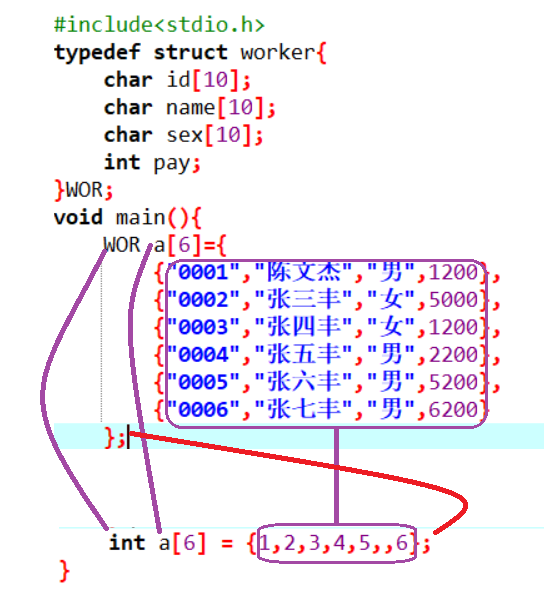结构体数组的输出
#include<stdio.h>
typedef struct worker{
char id;
char name;
char sex;
int pay;
}WOR;
void main(){
WOR a={
{"0001","陈文杰","男",1200},
{"0002","张三丰","女",5000},
{"0003","张四丰","女",1200},
{"0004","张五丰","男",2200},
{"0005","张六丰","男",5200},
{"0006","张七丰","男",6200}
};
int i;
for(i=0;i<6;i++){
printf("%s,%s,%s,%d\n",a[i].id,a[i].name,a[i].sex,a[i].pay);
}
}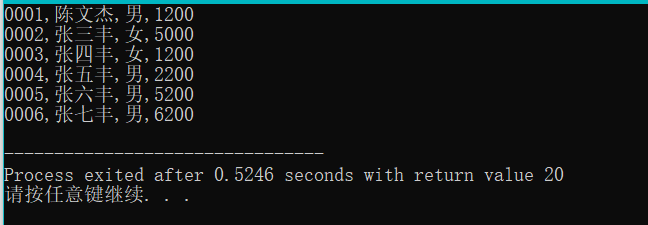结构体对象中的a[i]就代表一个对象，它已经不再是一个值了 所以我们应该用a[ii].成员名
改进以上程序
#include<stdio.h>
typedef struct worker{
char id;
char name;
char sex;
int pay;
}WOR;
void output(WOR a[],int n){//次函数是用来输出长度为n的工人数组的信息
int i;
printf("工号\t姓名\t性别\t年龄\n");
for(i=0;i<6;i++){
printf("%s\t%s\t%s\t%d\n",a[i].id,a[i].name,a[i].sex,a[i].pay);
}
}
void main(){
WOR a={
{"0001","陈文杰","男",1200},
{"0002","张三丰","女",5000},
{"0003","张四丰","女",1200},
{"0004","张五丰","男",2200},
{"0005","张六丰","男",5200},
{"0006","张七丰","男",6200}
};
output(a,6);
}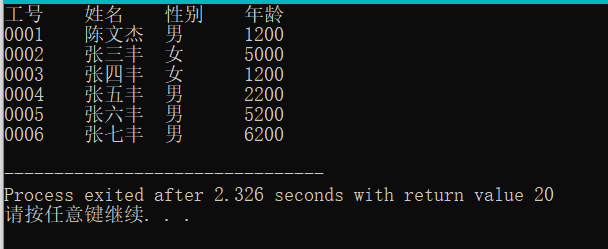结构体的应用
案例一、
1、编写一个函数，它的目的是为了输出所有性别为男的的职工信息 算法分析： 1)这个函数它不用返回值因为该函数是为了完成输出 2）该函数要处理很多职工，就需要一个数组，数组的类型为职工类型 注意：在C语言当中字符串不能直接比较是否相同，要通过strcmp这个函数来比较其两个字符串是否相同
#include<stdio.h>
#include<string.h>
typedef struct worker{
char id;
char name;
char sex;
int pay;
}WOR;
void QueryWorker(WOR a[],int n){//次函数是用来输出长度为n的工人数组的信息
int i;
printf("全部员工：\n");
printf("工号\t姓名\t性别\t年龄\n");
for(i=0;i<6;i++){
printf("%s\t%s\t%s\t%d\n",a[i].id,a[i].name,a[i].sex,a[i].pay);
}
printf("所有男员工：\n");
printf("工号\t姓名\t性别\t年龄\n");
for(i=0;i<6;i++){
if(strcmp(a[i].sex,"男")==0){
printf("%s\t%s\t%s\t%d\n",a[i].id,a[i].name,a[i].sex,a[i].pay);
}
}
printf("所有女员工：\n");
printf("工号\t姓名\t性别\t年龄\n");
for(i=0;i<6;i++){
if(strcmp(a[i].sex,"女")==0){
printf("%s\t%s\t%s\t%d\n",a[i].id,a[i].name,a[i].sex,a[i].pay);
}
}
}
void main(){
WOR a={
{"0001","陈文杰","男",1200},
{"0002","张三丰","女",5000},
{"0003","张四丰","女",1200},
{"0004","张五丰","男",2200},
{"0005","张六丰","男",5200},
{"0006","张七丰","男",6200}
};
QueryWorker(a,6);
}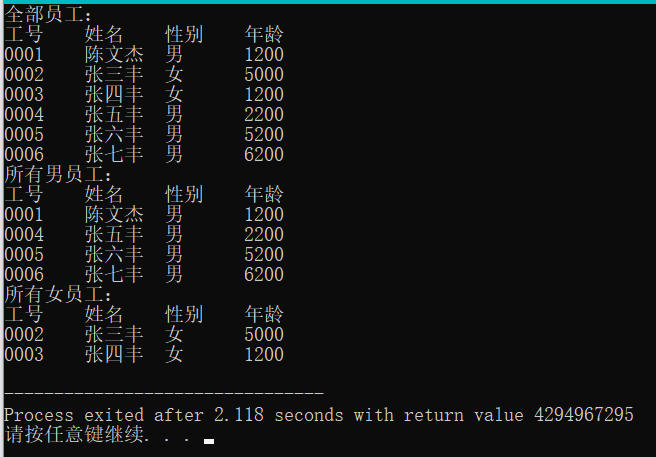2、能够输出指定性别的职工
#include<stdio.h>
typedef struct worker{
char id;
char name;
char sex;
int pay;
}WOR;
void QueryBySex(WOR a[],int n,char b[]){//次函数是用来输出长度为n的工人数组的信息
int i;

printf("工号\t姓名\t性别\t年龄\n");
for(i=0;i<6;i++){
if(strcmp(a[i].sex,b)==0){
printf("%s\t%s\t%s\t%d\n",a[i].id,a[i].name,a[i].sex,a[i].pay);
}
}

}
void main(){
WOR a={
{"0001","陈文杰","男",1200},
{"0002","张三丰","女",5000},
{"0003","张四丰","女",1200},
{"0004","张五丰","男",2200},
{"0005","张六丰","男",5200},
{"0006","张七丰","男",6200}
};
char b;
printf("请输入您要查询的性别 :\n");
scanf("%s",b);
QueryBySex(a,6,b);
}

案例二、
让用户输入一个工号，然后在一个结构体数组当中去查找有没有这个工人，如果有则输出这个工人的信息，如果没有则找不到此人
#include<stdio.h>
typedef struct worker{
char id;
char name;
char sex;
int pay;
}WOR;

void FindById(WOR a[],int n,char id[]){
//我们要访问数组里面每一个工人，如果发现了一个工人，就退出循环。如果找不到就输出找不到此人
int i;
for(i=0;i<n;i++){
if(strcmp(a[i].id,id)==0){//说明第i个工人就是我们要找的职工
break;//强制终止循环
}
}

//在退出循环之后，再来检查i与n的关系
if(i<n){//说明循环在中途就break,发现了职工
printf("%s\t%s\t%s\t%d\n",a[i].id,a[i].name,a[i].sex,a[i].pay);
}
else//找不到职工
{
printf("找不到此人");
}
}

void main(){
WOR a={
{"0001","陈文杰","男",1200},
{"0002","张三丰","女",5000},
{"0003","张四丰","女",1200},
{"0004","张五丰","男",2200},
{"0005","张六丰","男",5200},
{"0006","张七丰","男",6200}
};
char b;
printf("请输入您要查询的id :\n");
gets(b);
FindById(a,6,b);
}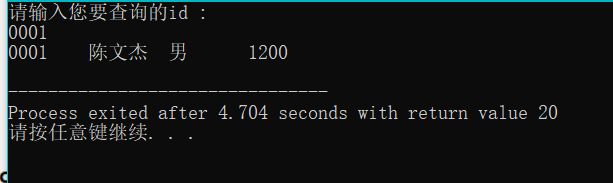案例三、
求出所有职工中最高工资那个职工的信息 注意：这里有一个知识点：成员比较整体赋值
#include<stdio.h>
#include<string.h>
typedef struct worker{
char id;
char name;
char sex;
int pay;
}WOR;
void max(WOR a[],int n){
WOR m=a;
int i;
for(i=0;i<n;i++){
if(m.pay<a[i].pay){//如果m的工资小于a[i]的工资，就把m赋值给a[i]
m=a[i];
}
}
printf("%s\t%s\t%s\t%d\n",m.id,m.name,m.sex,m.pay);
//假设第一个工人是最高工资的那个工人
}
void main(){
WOR a={
{"0001","陈文杰","男",1200},
{"0002","张三丰","女",7000},
{"0003","张四丰","女",1200},
{"0004","张五丰","男",2200},
{"0005","张六丰","男",5200},
{"0006","张七丰","男",6200}
};
max(a,6);
}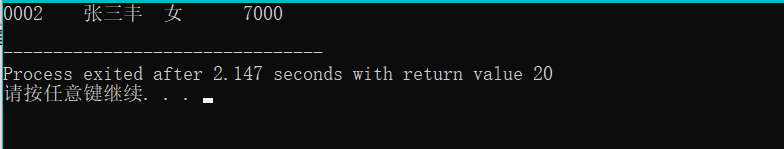上方程序改进 输出所有工资大于平均工资的职工 1、必须要先求出平均 2、在访问每一个职工，如果发现这个职工的工资要小于平均工资的话，就输出这个职工的信息。
#include<stdio.h>
#include<string.h>
typedef struct worker{
char id;
char name;
char sex;
int pay;
}WOR;
void QueryByAver(WOR a[],int n){
int i;
float aver;
int sum = 0;
//求出总工资
for(i=0;i<n;i++){
sum+=a[i].pay;
}
//求出平均工资aver
aver =(float)sum/n;
printf("平均工资为%f\n",aver);
printf("大于平均工资为：\n");
//访问每一个职工
for(i=0;i<n;i++){
if(a[i].pay>=aver){//如果发现职工的工资大于等于平局工资，就输出对应职工的信息
printf("%s\t%s\t%s\t%d\n",a[i].id,a[i].name,a[i].sex,a[i].pay);
}
}
}
void main(){
WOR a={
{"0001","陈文杰","男",3200},
{"0002","张三丰","女",7000},
{"0003","张四丰","女",1200},
{"0004","张五丰","男",2200},
{"0005","张六丰","男",5200},
{"0006","张七丰","男",6200}
};
QueryByAver(a,6);
}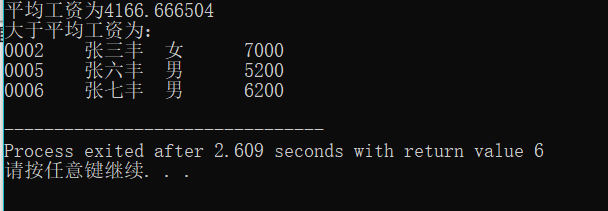算法变换
#include<stdio.h>
#include<string.h>
typedef struct stud{//定义了一个结构体
char Id;//学号
char Name;//姓名
int China;//语文成绩
int Math;//数学成绩
int English;//英语成绩
}STU;
//编写一个函数，它的目的是为了输出所有学生的总分与平均分
void fun(STU a[],int n){
int sum=0,i;
printf("学号\t姓名\t语文\t数学\t英语\t总分\t平局分\n");
//要访问里面的每一个学生
for(i=0;i<n;i++){
//对于一个学生a[i]而言我们要来输出它的信息
printf("%s\t%s\t%d\t%d\t%d\t%d\t%.1f\n",
a[i].Id,a[i].Name,a[i].China,a[i].Math,a[i].English,
a[i].China+a[i].Math+a[i].English,
(a[i].China+a[i].Math+a[i].English)/3.0);
sum = a[i].China+a[i].Math+a[i].English;
}
}
void main(){
STU a={
{"S1","张一军",127,150,100},
{"S2","张二军",75,85,89},
{"S3","张三军",67,58,86},
{"S4","张四军",120,101,87},
{"S5","张五军",136,100,82},
{"S6","张六军",141,52,83},
{"S7","张七军",125,85,81},
{"S8","张八军",136,65,79}
};
fun(a,8);
}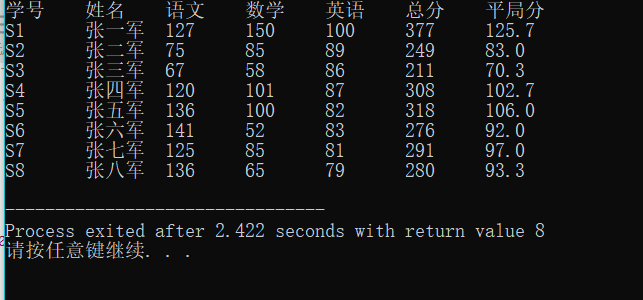案例变换： 注意字符串也不能够直接赋值，要通过一个函数来对字符串进行赋值 strcpy:通过这个函数才可以给字符串进行赋值
#include<stdio.h>
#include<string.h>
typedef struct stud{//定义了一个结构体
char Id;//学号
char Name;//姓名
int China;//语文成绩
int Math;//数学成绩
int English;//英语成绩
}STU;
//编写一个函数，求出每一个学生的优秀科目，目的不是输出分数，而是要输出对应科目的名称
void Perferct(STU a[],int n){
int sum=0,i;
char per;
int maxscore;//用来确保最优科目的分数
//要输出标题
printf("学号\t姓名\t语文\t数学\t英语\t最优秀科目\n");
//要访问里面的每一个学生，对于第i学生要确认它的最优科目名称（语文，数学，英语）
//科目名称是字符串，所以要先定义一个字符串来确保保存最优科目名称
for(i=0;i<n;i++){
maxscore=a[i].China;//认为第i个学生的语文是最优秀的
strcpy(per,"语文");
if(maxscore<a[i].Math){
maxscore = a[i].Math;
strcpy(per,"数学");
}
if(maxscore<a[i].English){
maxscore = a[i].Math;
strcpy(per,"英语");
}
printf("%s\t%s\t%d\t%d\t%d\t%s\n",
a[i].Id,a[i].Name,a[i].China,a[i].Math,a[i].English,per);
}
}

void main(){
STU a={
{"S1","张一军",127,150,100},
{"S2","张二军",75,85,89},
{"S3","张三军",67,58,86},
{"S4","张四军",120,101,87},
{"S5","张五军",136,100,82},
{"S6","张六军",141,52,83},
{"S7","张七军",125,85,81},
{"S8","张八军",136,65,79}
};
Perferct(a,8);
}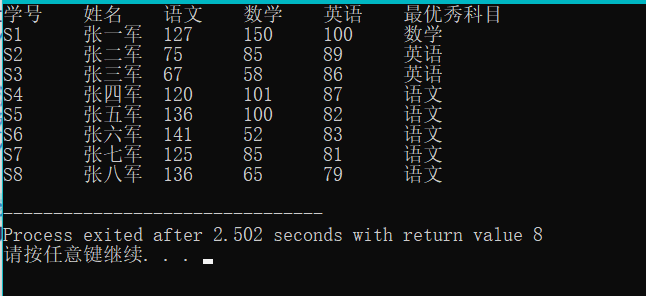展开全文c语言 程序设计 指针 编程语言
• //这里的1000可换成其他任意数，为该结构体数组最大容量 int main() { int i; int n; //n应小于其结构体数组最大容量 printf("请输入学生人数："); scanf_s("%d",&n); //输入学生信息 FILE *fp;
#include<stdio.h>
struct student
{
//学号
int num;
//姓名
char name;
//性别
char sex;
//分数
float score;
}stu;
//这里的1000可换成其他任意数，为该结构体数组最大容量

int main()
{
int i;
int n;

//n应小于其结构体数组最大容量
printf("请输入学生人数：");
scanf_s("%d",&n);

//输入学生信息
FILE *fp;
//这里的1000可换成其他任意数，为该结构体数组最大容量
fopen_s(&fp, "C:\\Users\\Asus\\Desktop\\demo_computer1.txt", "wt+");
for (i = 0; i < n && n <= 1000; i++)
{
printf("学号：");
scanf_s("%d", &stu[i].num);
getchar();
printf("姓名：");
gets_s(stu[i].name);

printf("性别：");
scanf_s("%c", &stu[i].sex);
printf("成绩：");
scanf_s("%f", &stu[i].score);
}
//保存到TXT中，以便下次该程序系统能读取其中学生信息
fwrite(stu, n * sizeof(struct student), 1, fp);
fclose(fp);

//保存到TXT中，供人们阅读其中学生信息
fopen_s(&fp, "C:\\Users\\Asus\\Desktop\\demo_people1.txt", "wt+");
for (i = 0; i < n && n <= 1000; i++)
{
fprintf(fp,"学号：%d\n", stu[i].num);

fprintf(fp,"姓名：%s\n",stu[i].name);

fprintf(fp, "性别：%c\n", stu[i].sex);

fprintf(fp, "成绩：%f\n\n", stu[i].score);
}
fclose(fp);

//读取TXT中信息并赋值给结构体数组
fopen_s(&fp, "C:\\Users\\Asus\\Desktop\\demo_computer1.txt", "rt+");

//显示所读取的学生信息
for (i = 0; i < n && n <= 1000; i++)
{
}
fclose(fp);

return  0;

}
展开全文• C语言结构体数组+结构体类型指针+指向结构体数组的指针+typedef类型结构体数组两种结构体数组的定义形式用sizeof运算符求结构体数组所占字节数结构体类型指针指向结构体数组的指针typedef类型 结构体数组 如果要处理...
• 1 #include <stdio.h> 2 #include <stdlib.h> 3 4 struct student{ 5 int num; 6 char str; 7 double dec; 8 }; 9 10 11 int main(){ 12 13 int n...有问必答 c++ c语言
• ## C语言--结构体数组和结构体指针

万次阅读 多人点赞 2018-08-16 21:06:49
1.结构体数组 具有相同结构体类型的变量构成的数组，称为结构体数组。与普通数值型数组的不同之处在于每个数组元素都是一个结构体变量，它们都分别包含各个成员项。 定义结构体数组一般形式是 struct 结构体名 { ...
• 示例代码： #include"stdio.h" #include"stdint.h" ...struct jiegouti//这是个结构体 { int8_t paraone;//第一个参数 uint8_t paratwo;//第二个参数 uint16_t parathree;//第三个参数 hansh...C语言 小白C语言 汉语拼音
• 结构体数组存储学生信息，输入学生学号，查询相应的成绩 主要是熟练一下结构体的运用，思路：先存储学生信息，然后做相应的查询，在存储过程中，设置以#为结束标志，在查找过程中，同样以#为结束标志，这里用到了...
• 定义一个职工结构体数组，从键盘输入5位职工信息，打印输出最高的工资。是一个很好的关于学习C语言结构体的例子。
• C语言中 结构体数组用 for循环和scanf输入 比应输入行数多一行，这是为什么？ 3组学生信息的输入，成绩汇总，排序，打印 我只想输3组数据（aa,bb,cc），但必须输4行(多了dd，这行是随便输的)才行，，不懂...c语言C 排序
• 学习使用C语言，并熟练掌握其操作过程。用数组结构体实现保存文件，录入内容为所有同学的学号、姓名、语文成绩和数学成绩。c++
• 结构体数组录入信息 简易版本，长度不变 #include<stdio.h> struct student { char name; char ID; int score;//五门课成绩 int sum; }stu; int main() { int i,j; for(i=0;i<3;i++) ...
• 1.定义结构体数组 和定义结构体变量类似，定义结构体数组时只需声明其为数组即可。如： 代码如下:struct Student{ int num; char name; char sex; int age; float score; char addr;};Student stu...
• C#:利用结构体数组完成简单信息输入、计算 using System; using System.Collections.Generic; using System.Linq; using System.Text; using System.Threading.Tasks; namespace norm_program { class Program { ...c#
• 如果使用gets(Math->name)就不会报错，但结构体成员的引用方式可以是"结构体变量名 . 成员名"啊。 如果使用gets(Math->name)也有问题! [图片说明]...图片 结构
• C语言从文件中读取一个结构体数组和把修改后的结构体数组存入到一个文件中 本人基础知识不在解释，帖子是我自己编的一个例子，很好理解，尤其是对初学C语言的童鞋，希望大家可以从中了解到用C语言从文件中读取一个...C语言 文件
• ## C++ 使用结构体数组

千次阅读 2019-08-01 17:02:20
1、结构体数组的使用 #include<iostream> using namespace std; #include<string> //创建一个学生的结构体 struct student { //成员列表 string name; int age; int score; }; int main() { //...
• ## 结构体&结构体数组

万次阅读 多人点赞 2018-08-16 18:17:39
1、结构体的定义 struct 结构体类型名 ｛ 类型名 成员名; 类型名 成员名; …… ｝; 先声明结构体类型，再定义结构体变量名 声明结构体类型，不分配空间 定义结构体类型变量，就要分配内存空间 1）结构体被...C
• ## 结构体和结构体数组

千次阅读 多人点赞 2020-03-14 11:14:21
一组数组异质的数组，但自成整体的数据 结构体变量的定义方法: 先声明结构体类型，再定义变量类型。 声明结构体变量时不分配空间，在定义结构体变量时才分配内存空间。 例1 学校体育考试，Tom得了一个不错...
• 对于结构体数组，前些天有个同事说链表真好用，他的关注点是插入排序(用于对蓝牙设备顺序存入，然后将新的蓝牙设备按照接收灵敏度插入到链表中，既完成了存入，又完成了排序)。因为他是第一次用链表，表述成了链表...c语言 开发语言 后端
• //定义结构体数组   struct Student stu = { {20154511, "liming", 'M', 99}, {20153424, "xiaowang", 'M', 66.5}, {20153623, "xiaohong", 'F', 59.5} };   struct Student *p;      //...指针
• ifstream 该数据类型表示输入文件流，用于从文件读取信息。 fstream 该数据类型通常表示文件流，且同时具有 ofstream 和 ifstream 两种功能，这意味着它可以创建文件，向文件写入信息，从文件读取信息。 一个简单的...
• 学生信息管理系统中可以用到结构体数组进行数据的输入输出 typedef struct student//定义结构体 { char stu_number; char stu_name; float usual_mark; float exam_mark; float overall_mark; }Student...c语言
• 【要求】 （1）定义结构体类型 （2）使用结构体数组，保存学生的信息 #include struct student { int num; char name; float s; }; int main() { int i, j=0; float max; struct student stu; for (i = 0; i...c语言
• //文件中存放了20个由小到大的排列的整数，现在从键盘上输入一个数，要求把该数插入此文件中，保持文件特性不变。 //基本思想是：先从文件中读取那20个数放入字符数组中，然后再对字符数组进行操作 #include<......